## We are designing a crude propulsion mechanism for a science fair demonstration. One of our team members stands on a skateboardthat has arigi

Question

We are designing a crude propulsion mechanism for a science fair demonstration. One of our team members stands on a skateboardthat has arigid“sail” attached. The other team members will throw object at the sail to propel the skateboard and rider. They have balls and globs of clay that are the same size and have the same mass. Will they have better results throwing the clay or the balls? Explain!

in progress 0
4 weeks 2021-08-30T21:36:55+00:00 1 Answers 0 views 0

greater speed will be obtained for the elastic collision,

Explanation:

To answer this exercise we must find the speed that the sail acquires after each impact.

Let’s start by hitting a ball of clay.

The system is formed by the candle and the clay balls, therefore the forces during the collision are internal and the moment is conserved.

initial instant. before the crash

p₀ = m v₀

where m is the mass of the ball and vo its initial velocity, we are assuming that the candle is at rest

final instant. After the crash

the mass of the candle is M

p_f = (m + M) v

the moment is preserved

p₀ = p_f

m v₀ = (m + M) v

v =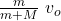for when n balls have collided

v =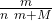v₀

Now let’s analyze the case of the bouncing ball (elastic)

initial instant

p₀ = m v₀

final moment

p_f = m v_{1f} + M v_{2f}

p₀ = p_f

m v₀ = m v_{1f} + M v_{2f}

m (v₀ – v_{1f}) = M v_{2f}

this case corresponds to an elastic collision whereby the kinetic energy is conserved

K₀ = K_f

½ m v₀² = ½ m v_{1f}² + ½ M v_{2f}²

v₁ = v_{1f}            v₂ = v_{2f}

m (v₀² – v₁²) = M v₂²

let’s use the identity

(a² – b²) = (a + b) (a-b)

we write our equations

m (v₀ – v₁) = M v₂                       (1)

m (v₀ – v₁) (v₀ + v₁) = M v₂²

let’s divide these equations

v₀ + v₁ = v₂

Let’s look for the final speeds

we substitute in equation 1

m (v₀ – v₁) = M (v₀ + v₁)

v₀ (m -M) = (m + M) v₁

v₁ =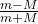v₀

we substitute in equation 1 to find v₂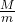v₂ = v₀ –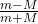v₀

v₂ =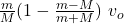v₂ =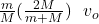v₂ =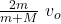Let’s analyze the results for inelastic collision with each ball that collides with the sail, the total mass becomes larger so the speed increase is smaller and smaller.

In the case of elastic collision, the increase in speed is constant with each ball since the total mass remains invariant.

Consequently, greater speed will be obtained for the elastic collision, that is, the ball will bounce.